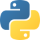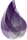The python elixir Euler project

This site will not help you cook pots of snakes potions. It is my diary as I make my way through the challenges at https://projecteuler.net/. I will attempt to use mathematics, as well as the programming languages python and elixir to solve them. There will be spoilers, so if you want to solve the problems yourself, stop right here!Solutions to the problems are not meant to be optimal or elegant, but rather a tool to continue learning

Problem 1

If we list all the natural numbers below 10 that are multiples of 3 or 5, we get 3, 5, 6 and 9. The sum of these multiples is 23.

Find the sum of all the multiples of 3 or 5 below 1000.

The sum up to $N$ of numbers which are multiples of 3 can be expressed as $S_3 = \sum_{n=1}^{N} 3n$. Equally for multiples of 5 up to $N'$ the sum will be: $S_5 = \sum_{n=1}^{N'} 5n$.

Or, more precisely the sum of multiples of 3 should go up to floor(1000/3)=333, since $333 \times 3 = 999$ will be the last multiple before $1000$. This however, will double-count some of the multiples. For example $\{15, 30, 45, 60\}$ are both in $S_3$ and $S_5$. We need to subtract the sum of the numbers which contain multiples of both which we can call $S_{3,5}$. This sum can be expressed as:

where $\lfloor \cdot \rfloor$ stands for the floor function. The sum of the first N numbers is equal to $\frac{N \times (N+1)}{2}$ , so our final answer will be:python

final_sum = 0for n in range(1,1000):    if n % 3 == 0 or n % 5:        final_sum += nprint(final_sum)# found in: 0.39ms

or in a more functional way:

xmultiples = filter(lambda x : x % 3 == 0 or x % 5 == 0, range(1,1000))final_sum = sum(list(multiples))print(final_sum)# found in: 0.21mselixir

xxxxxxxxxxismultiple = fn    (0, 0, _) -> true    (0, _, _) -> true    (_, 0, _) -> true    (_, _, n) -> false  end​keepit = fn (n) -> ismultiple.(rem(n, 3), rem(n, 5), n)  endEnum.filter(1..999, keepit)  |> Enum.sum  |> IO.puts# found in 1.15 ms